# How to annualize returns in Microsoft Excel

In this tutorial, we will learn how to annualize returns in Microsoft Excel. The annualization of returns in Excel is a commonly performed task that provides valuable insights. The general formula for annualizing returns is “((1+R)^n)-1“, with R representing the return rate and n representing the number of periods within one year.

Let’s say we have a data set that represents initial values, returned values, and the holding periods for each investment. We will calculate the annualized returns for each investment utilizing the following steps.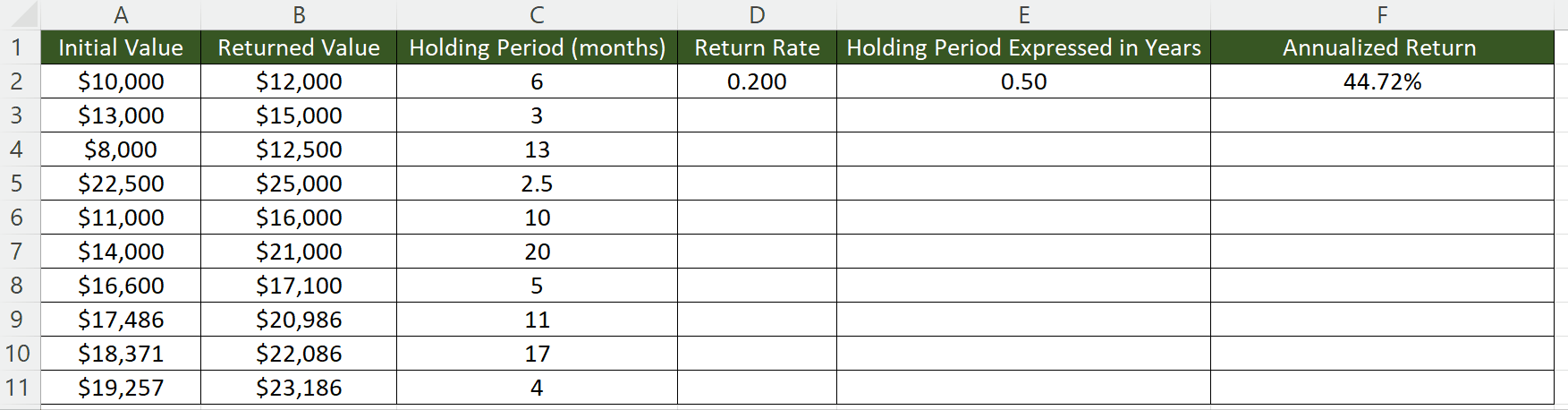Annualizing returns is a method that enables investors to compare the performance of various investments over diverse time frames on a yearly basis. Essentially, it offers a means of normalizing the returns of investments with varying holding periods, which aids investors in making better-informed investment decisions.

### Step 1 – Choose an Empty Cell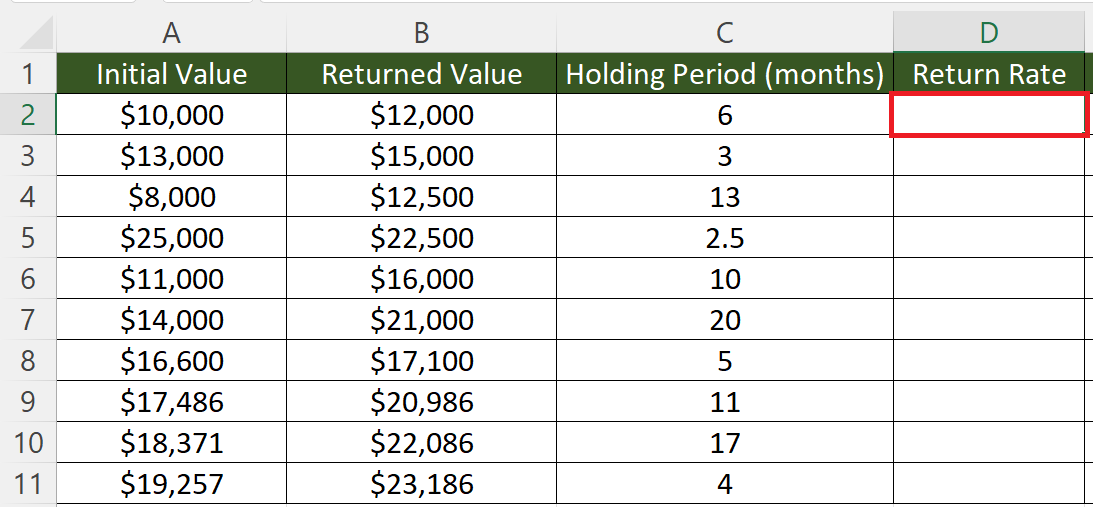– Choose an empty cell to calculate the return percentage.

### Step 2 – Calculate the Return Percentage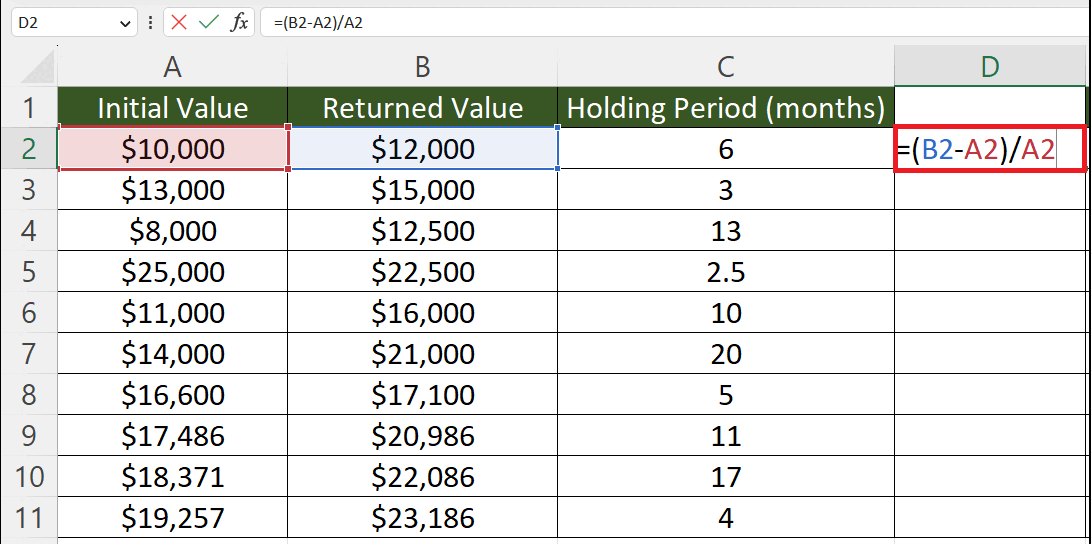– Calculate the return percentage by utilizing the formula:

### Step 3 – Convert the Holding Period into Years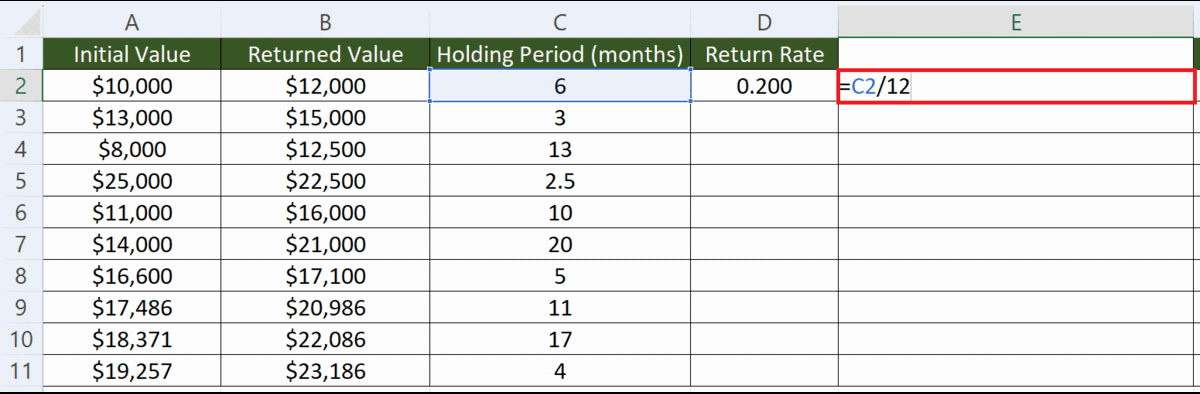– Choose an Empty cell.
– Convert the holding period into years i.e. in this case we have a holding period in months, we will divide it by 12 to get the holding period in years.

### Step 4 – Calculate the Annualized Returns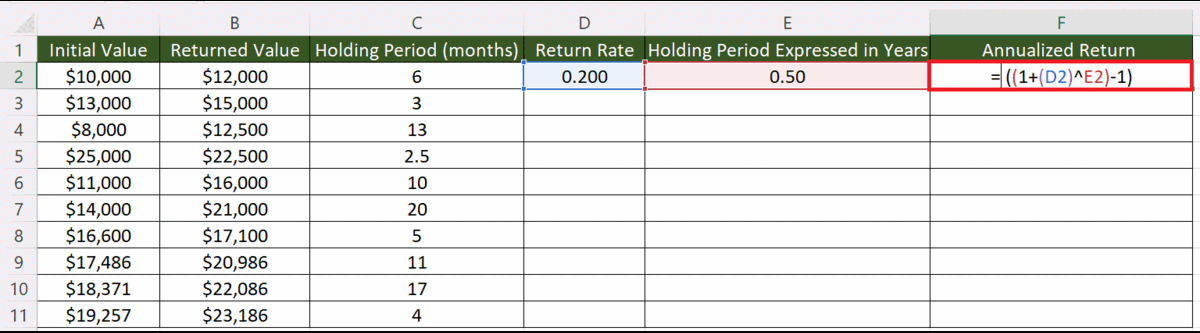– Calculate the annualized returns.
– The structure of the formula would be:
((1+(D2)^E2)-1)
– Where cell D2 contains the rate of return and cell F2 contains the holding period in years.

### Step 5 – Apply the Percentage Format to the Annualized Returns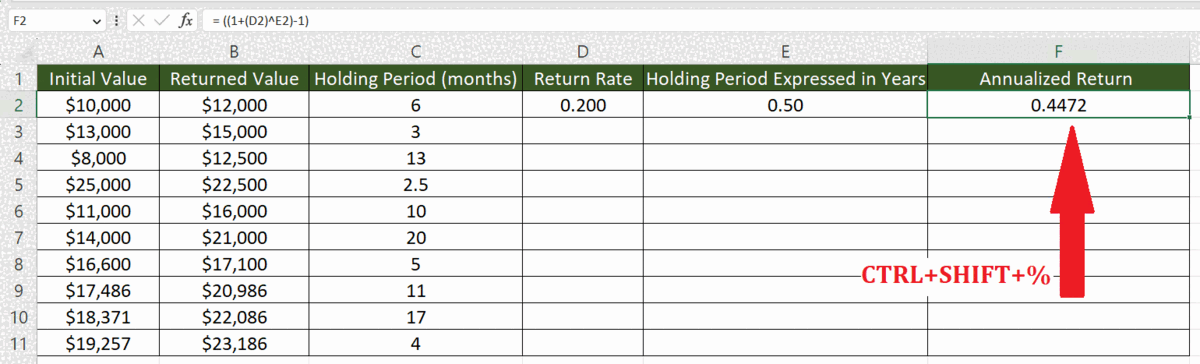– Apply the percentage format to the annualized returns.
– For this, select the cell and press CTRL+SHIFT+%.

### Step 6 – Utilize the Autofill Feature to Calculate the Annualized Return of Each– Utilize Autofill to calculate the annualized return for each value.